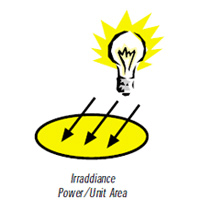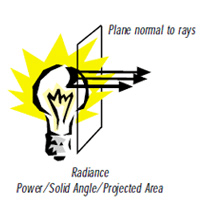#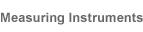# Language of Light

## 2.Concepts

Radiometry is the science of the measurement of electromagnetic (EM) radiation. The broader spectrum covered by the science of radiometry is based on physical constants.
The properties of concern to us here are radiated power and its spatial and angular distributions.
The four basic concepts are:

It is defined as the directed angular density of radiation from a source.The radiant intensity in a given direction is the sum of the power contained in all the rays (cones) emitted in that direction by the entire source (i.e., power per solid angle).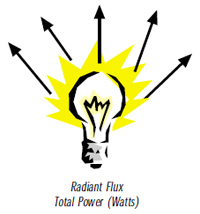This is the total radiant power emitted from a source or received by a surface. It can also be defined as the rate of flow of radiant energy through a certain area or out of a certain solid angle.
The SI unit of radiant flux is the Watt.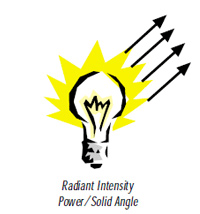This is a measure of radiant flux incident on an object’s surface (radiant flux per unit area).
The SI units for irradiance is Watt/square meter (Watt/m2)